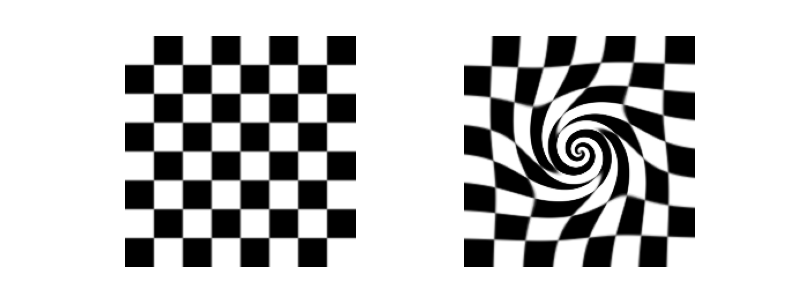# Swirl#

Image swirling is a non-linear image deformation that creates a whirlpool effect. This example describes the implementation of this transform in skimage, as well as the underlying warp mechanism.

## Image warping#

When applying a geometric transformation on an image, we typically make use of a reverse mapping, i.e., for each pixel in the output image, we compute its corresponding position in the input. The reason is that, if we were to do it the other way around (map each input pixel to its new output position), some pixels in the output may be left empty. On the other hand, each output coordinate has exactly one corresponding location in (or outside) the input image, and even if that position is non-integer, we may use interpolation to compute the corresponding image value.

## Performing a reverse mapping#

To perform a geometric warp in skimage, you simply need to provide the reverse mapping to the skimage.transform.warp() function. E.g., consider the case where we would like to shift an image 50 pixels to the left. The reverse mapping for such a shift would be:

def shift_left(xy):
xy[:, 0] += 50
return xy


The corresponding call to warp is:

from skimage.transform import warp
warp(image, shift_left)


## The swirl transformation#

Consider the coordinate $$(x, y)$$ in the output image. The reverse mapping for the swirl transformation first computes, relative to a center $$(x_0, y_0)$$, its polar coordinates,

\begin{align}\begin{aligned}\theta = \arctan((y-y0)/(x-x0))\\\rho = \sqrt{(x - x_0)^2 + (y - y_0)^2},\end{aligned}\end{align}

and then transforms them according to

\begin{align}\begin{aligned}r = \ln(2) \, \mathtt{radius} / 5\\\phi = \mathtt{rotation}\\s = \mathtt{strength}\\\theta' = \phi + s \, e^{-\rho / r} + \theta\end{aligned}\end{align}

where radius indicates the swirl extent in pixels, rotation adds a rotation angle, and strength is a parameter for the amount of swirl. The transformation of radius into $$r$$ is to ensure that the transformation decays to $$\approx 1/1000^{\mathsf{th}}$$ within the specified radius.import matplotlib.pyplot as plt

from skimage import data
from skimage.transform import swirl

image = data.checkerboard()
swirled = swirl(image, rotation=0, strength=10, radius=120)

fig, (ax0, ax1) = plt.subplots(nrows=1, ncols=2, figsize=(8, 3),
sharex=True, sharey=True)

ax0.imshow(image, cmap=plt.cm.gray)
ax0.axis('off')
ax1.imshow(swirled, cmap=plt.cm.gray)
ax1.axis('off')

plt.show()


Total running time of the script: ( 0 minutes 0.147 seconds)

Gallery generated by Sphinx-Gallery